# Poly-vector

(diff) ← Older revision | Latest revision (diff) | Newer revision → (diff)
Jump to: navigation, search-vector, over a vector spaceAn element of the-th exterior degree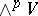of the spaceover a field(see Exterior algebra). A-vector can be understood as a-times skew-symmetrized contravariant tensor on. Any linearly independent system of vectorsfromdefines a non-zero-vector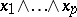; such a poly-vector is called factorable, decomposable, pure, or prime (often simply a poly-vector). Here two linearly independent systemsand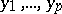generate the same subspace inif and only if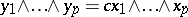, where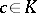. For any non-zero poly-vector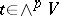, its annihilator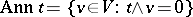is a subspace of dimension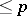, and the poly-vectoris pure if and only if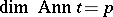. The pure-vectors of an-dimensional spaceform an algebraic variety in; the corresponding projective algebraic variety is a Grassmann manifold. Any non-zero-vector or-vector in an-dimensional spaceis pure, but a bivectoris pure if and only if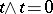.

Ifis a basis ofand, then the coordinates of the poly-vector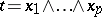in the basisof the spaceare the minors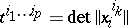,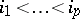, of the matrix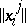. In particular, for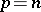,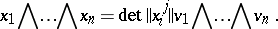If one specifies a non-zero-vector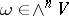, a duality between-vectors and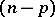-vectors is obtained, i.e. a natural isomorphism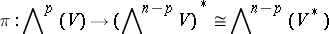such thatfor alland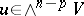.

Letand let an inner product be defined in, then inan inner product is induced with the following property: For any orthonormal basisinthe basis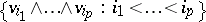inis also orthonormal. The scalar square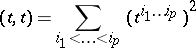of a pure poly-vectorcoincides with the square of the volume of the parallelopipedon inconstructed on the vectors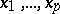. If one specifies an orientation in the-dimensional Euclidean space(which is equivalent to choosing an-vectorfor which), then the above duality leads to a natural isomorphism. In particular, the-vector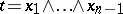corresponds to a vector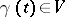, called the vector product of the vectors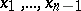.

How to Cite This Entry:
Poly-vector. Encyclopedia of Mathematics. URL: http://encyclopediaofmath.org/index.php?title=Poly-vector&oldid=17085
This article was adapted from an original article by A.L. Onishchik (originator), which appeared in Encyclopedia of Mathematics - ISBN 1402006098. See original article